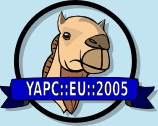# Mathematical Expression Handling With Perl

By Jonathan Worthington
Date: Thursday, 1 September 2005 17:40
Duration: 20 minutes
Language:

I have been doing some work on how to store and enable easy manipulation of
mathematical expressions in Perl. The talk would look at this topic,
demonstrating some CPAN modules that I have authored relating to this area
and showing how using a different internal representation for something can
greatly simplify its manipulation, a principle more widely applicable. I
will also show how reducing expressions to a representation using basis
function might provide a way of demonstrating the equivalence of two
expressions, something I'm currently working on.

Note that some of this was covered in an article I wrote for "The Perl
Journal" in February 2005.

MAIN POINTS
* Why analytical manipulation instead of numerical manipulation might be
useful
* Forming an internal representation (in this case, a non-strict binary
tree)
* Introducing Math::Calculus::Expression
* How the internal representation makes manipulation easy
* Example of subclassing Math::Calculus::Expression to do various bits of
manipulation
* Testing expression equivalence by looking to representation in basis
functions